# Team:Aberdeen Scotland/Curve Fitting

University of Aberdeen - ayeSwitch - iGEM 2010

iGEM 2010

# Determination of the the Hill Coefficient of the CFP/MS2 loop Association (n2)

Based on a graph in a paper by Witherell et al. which showed the binding curves of the MS2 stem loop we could calculate more accurately the value for n2. The initial estimate for n2 was between 1 and 3 (see Parameter Space Analysis). Our two MS2 stem loops (see Fig 1 in Equations) are 19 nucleotides apart, so our binding curve will most closely resemble that of the 8-16 construct, shown in figure 1A (filled squares).

Unfortunately we did not have a table of data available to us, so we had to estimate the values directly from the graph (figure 1A). These values were entered into MATLAB, and using MATLAB’s curve fitting tool we fit the Hill function for activators to the curve (figure 1B). The parameters for the best fit curve was then returned. However, the value returned is based on having one MS2 stem loop and we have two MS2 stem loops. Therefore, the value returned needs to be multiplied by two to give the final result for n2.

To recap, the equation for the Hill function for activators is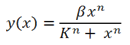, where β is equivalent to our λ values, x is the environmental signal (GAL or METH), K is the dissociation constant and n is the Hill coefficient.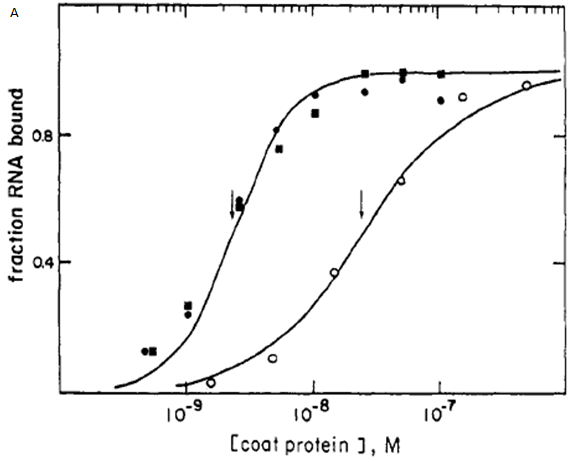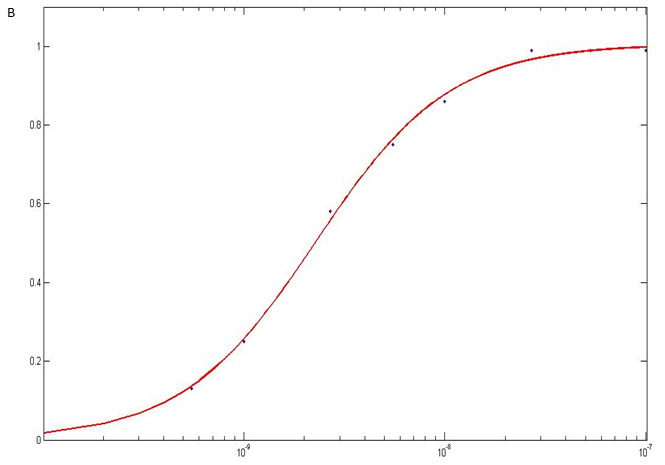Figure 1. A. Graph from paper by Witherell et al. showing the binding curves of the MS2 stem loop. The filled squares are the 8-16 construct
which closely resembles the binding curves of our MS2 stems. B. The binding curve for the 8-16 construct was reproduced in MATLAB and the Hill
function for activators equation fitted to it (red line).

The curve fitting tool gave the following estimated parameters for β(a), K(b) and n(c):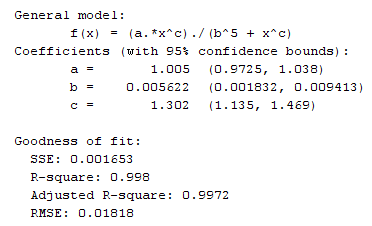Note that the R-square value is close to one which suggests that the fit of the curve to the data is very good.

The Hill coefficient is estimated to be 1.302 with a lower limit of 1.135 and an upper limit of 1.469. However, this is just for one MS2 stem loop and we have two. Multiplying this value by 2 we get 2.604 with a lower limit of 2.270 and an upper limit of 2.938. A value greater than 2 suggests that a protein binding to the first stem loop will make it easier for a protein to bind to the second stem loop. We can say that co-operativity has been increased.

### Conclusion

We have n2=2.6 and n4=1. So, the equations of our system can be written as follows: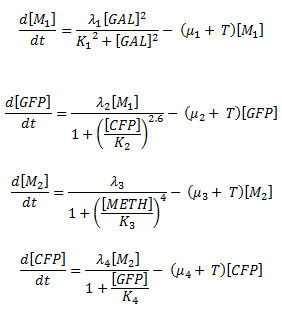Table 1 on the Parameter Space Analysis page shows that with n2=2.6 and n4=1, between 0.96% and 2.03% of the parameter combinations tested gave bistability.

However, the ideal scenario is that in table 4 on the Parameter Space Analysis page. Here, with n2=2.6 and n4=1, 51.27% to 58.04% of parameter combinations tested gave bistability.

The reason we are not getting bistability 100% of the time, or 0% of the time, is because we have such a large range around each parameter. Each parameter value is randomly chosen from this range each time the program runs. Therefore, some combinations of parameters will give bistability and others will not. Ideally we would have a precise value for each parameter (no uncertainty). In this scenario, each time the program runs the parameters would be exactly the same. The result would either be 100% bistability or 0% bistability – either the switch always works or it doesn’t.

### References

 Witherell, G.W., et al. (1990), ‘Cooperative Binding of R17 Coat Protein to RNA’, Biochemistry, Vol. 29, pp. 11051-11057

Back to the Top